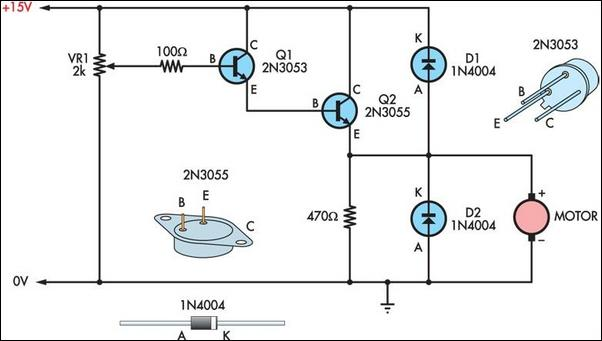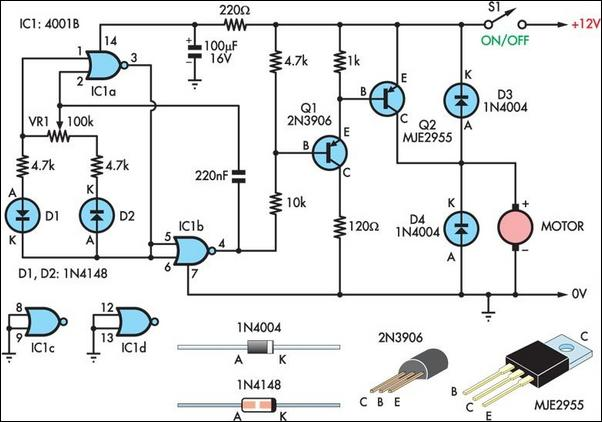# Learning Electronics

Learn to build electronic circuits

# Two Basic Motor Speed Controllers

Here are two simple 12V DC motor speed controllers that can be built for just a few dollars. They exploit the fact that the rotational speed of a DC motor is directly proportional to the mean value of its supply voltage. The first circuit shows how variable voltage speed control can be obtained via a potentiometer (VR1) and compound emitter follower (Q1 & Q2). With this arrangement, the motor’s DC voltage can be varied from 0V to about 12V. This type of circuit gives good speed control and self-regulation at medium to high speeds but very poor low-speed control and slow starts. The second circuit uses a switchmode technique to vary motor speed.

Circuit diagram:Fig.1: a very simple motor speed controller based on a compound emitter follower (Q1 & Q2).

Here a quad NOR gate (IC1) acts as a 50Hz astable multivibrator that generates a rectangular output. The mark-space ratio of the rectangular waveform is fully variable from 20:1 to 1:20 via potentiometer VR1. The output from the multivibrator drives the base of Q1, which in turn drives Q2 and the motor. The motor’s mean supply voltage (integrated over a 50Hz period) is thus fully variable with VR1 but is applied in the form of high-energy "pulses" with peak values of about 12V.

Circuit diagram:Fig.2: this slightly more complicated circuit gives better low speed control and higher torque.

This type of circuit gives excellent full-range speed control and gives high motor torque, even at very low speeds. Its degree of speed self-regulation is proportional to the mean value of the applied voltage. Note that for most applications, the power transistor (Q2) in both circuits will need to be mounted on an appropriate heatsink.
Author: Ravi Sumithraarachchi - Copyright: Silicon Chip Electronics